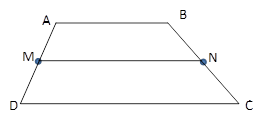Chapter 4.4, Problem 12E### Elementary Geometry for College St...

6th Edition
Daniel C. Alexander + 1 other
ISBN: 9781285195698

#### Solutions

Chapter
Section### Elementary Geometry for College St...

6th Edition
Daniel C. Alexander + 1 other
ISBN: 9781285195698
Textbook Problem
1 views

# In Exercises 11 to 16, the drawing shows trapezoid ABCD with A B ¯ | | D C ¯ ; also, M and N are midpoints of A D ¯ and B C ¯ , respectively.Exercises 11-16Given: M N = 6.3   a n d   D C = 7.5 Find: A B

To determine

To Find:

AB of trapezoid ABCD.

Explanation

Consider the following trapezoid ABCD.

Given:

MN=6.3 and DC=7.5

In the given trapezoid, AB¯||DC¯.

M is the midpoint of the side AD¯ and N is the midpoint of the side BC¯

### Still sussing out bartleby?

Check out a sample textbook solution.

See a sample solution

#### The Solution to Your Study Problems

Bartleby provides explanations to thousands of textbook problems written by our experts, many with advanced degrees!

Get Started

#### A sample of n = 9 scores has X = 108. What is the sample mean?

Essentials of Statistics for The Behavioral Sciences (MindTap Course List)

#### Convert the expressions in Exercises 6584 to power form. x23

Finite Mathematics and Applied Calculus (MindTap Course List)

#### True or False: The slope of a tangent line may be interpreted as average velocity.

Study Guide for Stewart's Single Variable Calculus: Early Transcendentals, 8th

#### What are the five steps of the scientific method?

Research Methods for the Behavioral Sciences (MindTap Course List)